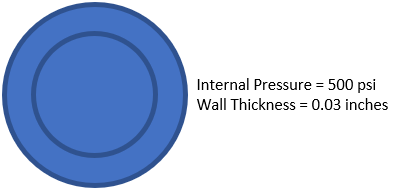## Hoop Stress

If a thin-walled cylinder pressure vessel in the figure below has an inner radius of 0.25”, what is the tangential (hoop) stress?Hint
Tangential (hoop) stress of a thin-walled cylinder:
$$\sigma_t=\frac{P_ir}{t}$$$where $$P_i$$ is the internal pressure, $$r$$ is the average radius, and $$t$$ is the wall thickness. Hint 2 $$r=\frac{r_i+r_o}{2}$$$
where $$r_i$$ is the inner radius, and $$r_o$$ is the outer radius.
Remember, a cylinder can be considered thin-walled if the wall thickness is about 1/10 or less of the inside radius. If so, the internal pressure is resisted by both the axial and hoop stress. Tangential (hoop) stress of a thin-walled cylinder:
$$\sigma_t=\frac{P_ir}{t}$$$where $$P_i$$ is the internal pressure, $$r$$ is the average radius, and $$t$$ is the wall thickness. To find $$r$$ : $$r=\frac{r_i+r_o}{2}$$$
where $$r_i$$ is the inner radius, and $$r_o$$ is the outer radius. Thus,
$$r=\frac{0.25inch+(0.25inch+0.03inch)}{2}=\frac{0.53inch}{2}=0.265\:inch$$$Therefore, $$\sigma_t=\frac{500\frac{lb}{in^2}(0.265in)}{0.03in}=\frac{132.5}{0.03}psi=4,417\:psi$$$
4,417 psi